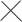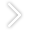# [分享]桥梁无支架现浇盖梁计算，很全很实用！01
PART1.1  荷载计算1.2 支架刚度计算1.3 承重钢棒强度计算02
PART2.1 荷载设计

2.2 钢带与墩柱的摩擦力计算a                                                               a
KG          1.2´722.4´1032.3 焊接设计03
PARTXX大桥最大的盖梁为C30钢筋砼，总方量为36.03m³，砼容重取25KN/m³。采用两根50a工字钢作为纵梁，间距1.6~2m，纵梁长12m，纵梁上布置14工字钢作为横梁，横梁长4m，间距为40cm，共31根。抱箍采用两块半圆形钢板制作，钢板厚12mm，高66cm，抱箍牛腿钢板厚20mm，宽35cm，采用30根M24的高强螺栓连接，为提高墩柱与抱箍之间的摩擦力，保护墩柱混凝土面，墩柱与抱箍之间设置3mm厚的橡胶垫。布置结构如图所示：1、荷载大小
⑴施工人员、机具、材料荷载取值：
P1=2.5KN/㎡
⑵混凝土冲击及振捣混凝土时产生的荷载取值：
P2=2.5KN/㎡
⑶盖梁钢筋混凝土自重荷载：
①变截面处：
P31=30.625KN/㎡
②均截面处：
P32=40KN/㎡
⑷模板支架自重荷载取值：
P4=1.5KN/㎡
2、I14工字钢受力检算
14工字钢的弹性模量E=2.1×105MPa，惯性矩I=712cm4，截面系数W=102 cm3，理论重量m=16.89kg/m，Q235钢的抗剪强度fv取85 MPa，抗弯强度fm取145MPa，则以单根横梁为例进行验算。
⑴荷载计算
①施工人员、机具、材料荷载：
q1=P1l=2.5×0.4=1KN/m
②混凝土冲击及振捣混凝土时产生的荷载：
q2=P2l=2.5×0.4=1KN/m
③盖梁钢筋混凝土自重荷载：
q31=P31l=30.626×0.4=12.25KN/m；q32=P32l=40×0.4=16KN/m
④模板、支架及横梁自重荷载
q4=P4l+ gk=1.5×0.4+0.17=0.77KN/m

（1+1）×1.4+（16+0.77）×1.2=22.924 KN/m

（1+1）×1.4+（12.25+0.77）×1.2=18.424KN/m

Mmax= ql2/8=22.924×2²/8=11.462KN.m,

ω=5ql4/384EI=5×22.924×16×1012/384×2.1×105×712×104=0.003mm＜[ω] =l/800=2.5mm，符合要求3、I50a工字钢受力检算
50a工字钢的弹性模量E=2.1×105MPa，惯性矩I=46500cm4，截面系数W=1860 cm3，理论重量m=93.654kg/m，Q235钢的抗剪强度fv取85 MPa，抗弯强度fm取145MPa，纵梁的跨距为7m，则以单根纵梁为例进行验算。
⑴荷载计算
①施工人员、机具、材料荷载：
q1=P1l=2.5×1.8=4.5KN/m
②混凝土冲击及振捣混凝土时产生的荷载：
q2=P2l=2.5×1.8=4.5KN/m
③盖梁钢筋混凝土自重荷载：
q31=P31l=30.626×1.8=55.125KN/m；q32=P32l=40×1.8=72KN/m
④模板、支架、横梁及纵自重荷载
q4=P4l+ gk=1.5×1.8+0.17×31+0.94=8.91KN/m

（4.5+4.5）×1.4+（72+8.91）×1.2=109.7KN/m

（4.5+4.5）×1.4+（55.125+8.91）×1.2=89.44KN/m

Mmax= ql2/24=109.7×7²/24=224KN.m,

ω=5ql4/384EI=5×224×2401×1012/384×2.1×105×4.65×108=0.07mm＜[ω] =l/800=8.75mm，符合要求4、抱箍的受力验算

⑴螺栓的允许承载力验算
[NL]=Pμn/K=225×0.3×1/1.7=39.71KN
⑵螺栓数目计算
m=N/[NL]=433.75/39.71=10.9≈12个，取计算截面上的螺栓数目30个，则每条高强螺栓提供的抗剪力：
P=N/m=433.75/30=14.46KN＜[NL]=39.71 KN，符合要求，能承担所要求的荷载。
⑶螺栓轴向受拉计算

N1= Pb=1435.83KN

N0= Pb/m=1435.83/30=47.86KN
σ=N/A= Pb（1-0.4m/n）/A=1435.83×（1-0.4×30/30）/4.5×30=63.8MPa＜[σ]=225 MPa，故高强螺栓满足强度要求。
⑷螺栓需要的力矩，μ1=0.15、L1=0.018

M2=（μ1N0cos8°+ N0sin8°）L2=0.165 KN.m
M= M1+ M2=0.129+0.165=0.294 KN.m，故螺栓的拧紧力矩M≥0.294 KN.m
⑸抱箍体的应力计算

S=0.012×0.66=0.00792㎡，则抱箍壁受到的拉应力为：
σ=P/S=717.9/0.008=87.74MPa＜[σ]=140MPa，符合要求。

04
PART1）预留孔：立柱施工时测好预留孔的标高位置，预埋直径110mm硬质PVC管，较高立柱根据高差来进行标高调整，保证两预留孔处于同一个标高，施工时把有关主筋间距和上下层箍筋间距作微调；
2）插入钢棒：柱顶插入一根直径为9cm，长度为300cm的钢棒，作为主梁工字钢支撑点，钢棒外伸长度一致；
3）安装固定装置和机械式千斤顶。
4）吊装主梁工字钢，利用φ25精轧螺纹钢，夹紧主梁工字钢，上铺I12.6工字钢作为分配梁；
5）拆除钢棒，封堵预留孔：盖梁施工完成后把预留孔用细石混凝土封堵。

1、设计参数
1）I12.6工字钢

2）主梁工字钢

3）钢棒

2、荷载计算
1) 混凝土自重荷载（考虑立柱混凝土重量）
W1=38.35×26=444.3kN；
2）支架、模板荷载
A、2片I45b组成主梁,长12m，纵向工字钢长4.5m，间距30cm。
W2=12×0.874×2+0.142×4.5×（11/0.3）=54.3kN；
B、定型钢模板,重量由厂家设计图查询得到。
W3=6800×10=68kN；
3）施工人员、机械重量。

W4=12×2×1=24kN；
4）振捣器产生的振动力。

5）荷载集度计算

2、强度、刚度计算
1) I12.6工字钢强度验算σ=M/Wx=6.2×1000/77×10-6=80.5MPa <[σ]=215MPa;

τ=Q/A=12.6×1000/18.1×10-4=6.96MPa <[σ]=125MPa;

2）主梁工字钢I45b计算σ= 103MPa <[σ]=215MPa;

τ=34MPa <[σ]=125MPa;

3）钢棒验算

τ=Q/A=288.4×1000/0.006362=45.3MPa <[σ]=125MPa

发表于2021-09-14   |  只看该作者       筑龙币+10

2

发表于2021-09-14   |  只看该作者

3

发表于2021-09-25   |  只看该作者

4# 大眼睫毛精

4 关注

999+ 粉丝

178 发帖

107 荣誉分

## 添加简介及二维码## 发站内信息2/20